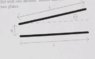# Calculating the capacitance of a system of two plates tilted at a small angle

In summary, the conversation discusses the calculation of capacitance for a system of two square plates separated by a distance D and making an angle θ with each other. The method involves breaking the system into infinitesimal strips and using the equation C=Kε0A/d to calculate the capacitance. However, the method used assumes a constant charge on each elemental capacitor, which is not correct. It is important to check the answer, as in this case, the capacitance should approach that of two parallel L x L plates when the angle approaches 0.

## Homework Statement

A capacitor is made up of a pair of square plates, each of side length L, that are very nearly parallel (such that θ is very small) and separated by a distance D. The plates make an angle, θ, with one another. The capacitor may be thought of as being comprised of many infitesimally think strips of width, dx, and length L, which are effectively in parallel with one another. Recall that sinθ≈θ for θ<<1rad. Calculate the capacitance of the system of two plates. Capacitor.jpg attached is a picture of the problem.

## Homework Equations

C=Kε0A/d, K=1(vacum)

## The Attempt at a Solution

C=Kε0A/d=ε0L2/d=ε0L2∫1/(Lsinθ+D)dL (integral lower limit 0 upper limit L)
C=ε0(LN(Lsinθ+D)-LN(0))
I believe this is right just wanted confirmation on my method...

#### Attachments

•Capacitor.jpg
6.2 KB · Views: 392
Last edited by a moderator:
A quick look at your answer and I see a problem ln(0) = ∞ .

••